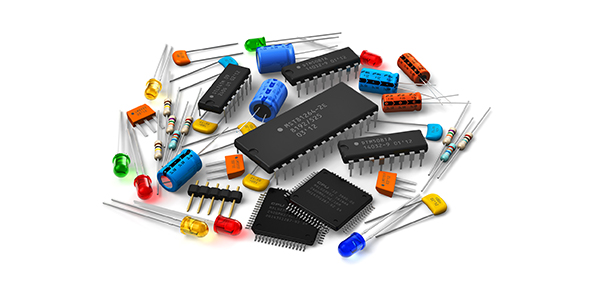# Quiz: Neets Module 1

30 Questions | Total Attempts: 6345SettingsNEETS_electronics reviewer

• 1.
Matter can be found in which of the following forms?
• A.

Solid

• B.

Liquid

• C.

Gaseous

• D.

Each of the above

• 2.
A substance that CANNOT be reduced to a simpler substance by chemical means is called a/an
• A.

Element

• B.

Mixture

• C.

Compound

• D.

Solution

• 3.
A molecule is the smallest possible particle that retains the characteristic of which of the following substances?
• A.

An element

• B.

A mixture

• C.

A compound

• D.

A solution

• 4.
What subatomic particle has a negative charge and a small mass?
• A.

Proton

• B.

Electron

• C.

Positron

• D.

Neutron

• 5.
What subatomic particle has a positive charge and a large mass?
• A.

Proton

• B.

Electron

• C.

Positron

• D.

Neutron

• 6.
What subatomic particle has no charge?
• A.

Proton

• B.

Electron

• C.

Positron

• D.

Neutron

• 7.
An atom is the smallest possible particle that retains the characteristic of which of the following substances?
• A.

An element

• B.

A mixture

• C.

A compound

• D.

A solution

• 8.
When light is represented as a tiny packet of energy, what are these packets of energy called?
• A.

Angstroms

• B.

Photons

• C.

Wavelengths

• D.

Frequencies

• 9.
If light energy collides with an orbiting electron, what happens to the electron?
• A.

The electron will move around the same orbit faster

• B.

• C.

• D.

The electron will merge with the nucleus

• 10.
The number of electrons in the outermost shell of an atom determines which of the following characteristics of the atom?
• A.

Valence

• B.

Atomic weight

• C.

Atomic number

• D.

Number of shells

• 11.
When an atom gains or loses an electron, which of the following terms applies?
• A.

Unbalanced

• B.

Lightened

• C.

Neutral

• D.

Ionized

• 12.
What is the main difference between conductors, semiconductors, and insulators?
• A.

The temperature differences

• B.

The physical state of their mass

• C.

The number of free electrons

• D.

The designations of the outer shells

• 13.
A substance with an excess of electrons is considered to be in what electrical state?
• A.

Neutral

• B.

Positive

• C.

Negative

• D.

Discharged

• 14.
Which of following actions describes the easiest way to accumulate a static electric charge?
• A.

Friction between two conductors

• B.

Friction between two insulators

• C.

Pressure between two conductors

• D.

Pressure between two insulators

• 15.
An atom that contains 6 protons and 5 electrons has what electrical charge?
• A.

Positive

• B.

Negative

• C.

Neutral

• D.

Intermediate

• 16.
How do "like" and "unlike" charges react to one another?
• A.

Unlike charges repel each other, like charges repel each other

• B.

Unlike charges attract each other, like charges attract each other

• C.

Unlike charges repel each other, like charges attract each other

• D.

Unlike charges attract each other, like charges repel each other

• 17.
What is/are the term(s) applied to the space between and around charged bodies in which their influence is felt?
• A.

Electric field of force

• B.

Electrostatic field

• C.

Dielectric field

• D.

Each of the above

• 18.
Electrostatic lines of force are drawn in which of the following manners?
• A.

Entering negative charge, entering positive charge

• B.

Entering negative charge, leaving positive charge

• C.

Leaving negative charge, leaving positive charge

• D.

Leaving negative charge, entering positive charge

• 19.
Which of the following devices use magnetism?
• A.

Batteries

• B.

Light bulbs

• C.

High-fidelity speakers

• D.

Each of the above

• 20.
Magnetic materials have which of the following qualities?
• A.

They are attracted by magnets

• B.

They can be magnetized

• C.

Both 1 and 2 above

• D.

They are electrical insulators

• 21.
Ferromagnetic materials have which of the following qualities?
• A.

They are all alloys

• B.

They all contain nickel

• C.

They make very weak magnets

• D.

They are relatively easy to magnetize

• 22.
A material with low reluctance and high permeability such as iron or soft steel is used to make what type of magnet?
• A.

Temporary

• B.

Permanent

• C.

Residual

• D.

Natural

• 23.
The ability of a material to retain magnetism is called
• A.

Permeability

• B.

Retentivity

• C.

Reluctance

• D.

Ionization

• 24.
The law of magnetic poles states which of the following relationships?
• A.

Like poles attract, unlike poles attract

• B.

Like poles attract, unlike poles repel

• C.

Like poles repel, unlike poles repel

• D.

Like poles repel, unlike poles attract

• 25.
The north indicating pole of a compass needle is attracted to which of the following poles of the earth?
• A.

The geographic north pole

• B.

The magnetic north pole

• C.

The geographic south pole

• D.

The magnetic south pole

Related TopicsBack to top